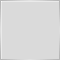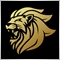11

Hi, I moved to new computer and was forced to install a new built of MT4 way above 600. Almost all my compiled scripts are working, but those that don't return the same error: "not all control paths  return a value". In theory I understnad what it might mean, but with my basic programming skills I can't find where exactly am I missing some cases and why before it was alright. for example this script below doesn't even have if-else in it and still returns the same error:

```double risk;

string lotall;
string pairM={"NZDUSD","AUDUSD","USDJPY","EURUSD"};
string lotM;
int i, j;
string mytext, mytextM;

int start()
{
risk = GlobalVariableGet("risk");

i=0;
mytext="With risk ="+DoubleToStr(risk,2)+"\r \r symbol      lot    spread";
while(i<ArrayRange(pairall,0))
{
lotall[i] = DoubleToStr(risk*AccountFreeMargin()/MarketInfo(pairall[i],MODE_MARGINREQUIRED),2);
i++;}

j=0;
mytextM="\r";
while(j<ArrayRange(pairM,0))
{
lotM[j] = DoubleToStr(risk*AccountFreeMargin()/MarketInfo(pairM[j],MODE_MARGINREQUIRED),2);
j++;}

MessageBox(mytext+mytextM,NULL,EMPTY);

}
```Moderator
2308

```double risk;

string lotall;
string pairM={"NZDUSD","AUDUSD","USDJPY","EURUSD"};
string lotM;
int i, j;
string mytext, mytextM;

int start()
{
risk = GlobalVariableGet("risk");

i=0;
mytext="With risk ="+DoubleToStr(risk,2)+"\r \r symbol      lot    spread";
while(i<ArrayRange(pairall,0))
{
lotall[i] = DoubleToStr(risk*AccountFreeMargin()/MarketInfo(pairall[i],MODE_MARGINREQUIRED),2);
i++;}

j=0;
mytextM="\r";
while(j<ArrayRange(pairM,0))
{
lotM[j] = DoubleToStr(risk*AccountFreeMargin()/MarketInfo(pairM[j],MODE_MARGINREQUIRED),2);
j++;}

MessageBox(mytext+mytextM,NULL,EMPTY);

return(0);
}```11

Wow, that's the only thing missing?! Thank you very much! I suppose I can fix that way all my scripts. Perfect!38701

Wow, that's the only thing missing?! Thank you very much! I suppose I can fix that way all my scripts. Perfect!
https://www.mql5.com/en/articles/1391#1_4109

bool orderprofit()

{
for(int i=OrdersTotal()-1;i>=0;i--)
{
{
return(True);
else
return(False);
}
}
}

but give me this error ""not all control paths  return a value""Moderator
12729

bool orderprofit()

{
for(int i=OrdersTotal()-1;i>=0;i--)
{
{
return(True);
else
return(False);
}
}
}

but give me this error ""not all control paths  return a value""

Because it doesn't return anything when orderselect fails.

```bool orderprofit()

{
for(int i=OrdersTotal()-1;i>=0;i--)
{
{
return(True);
}
}
return(False);
}```109

Marco vd Heijden:

Because it doesn't return anything when orderselect fails.

thanks